Start typing, then use the up and down arrows to select an option from the list.# Ksp Determination

Jules Bruno
149views
1
3
here. It states that a saturated solution of Lumen chloride contains 5.15 times 10 to negative for Mueller chloride ions. What is the salt ability product Constant or what's K SP? Since we're dealing with CSP here, we're dealing with aluminum chloride. We're dealing with its solid form. It breaks up into its eye on, so it breaks up into aluminum ion plus three Cloyd islands. We're dealing with a nice chart. So initial change equilibrium. Remember, in a nice chart, we ignore solids and liquids here. This is being broken down in pure water. So initially these two or zero their products or making them so this would be plus X. There's a three year so this would be plus three x plus X plus three X Now remember, K SP is just like all other cases equals products overreacting but are reacting will be a solid so ignore our reacted. So k spes equals products, therefore equals aluminum ion times chloride ion And again this three here means that this is to the third power here We're looking for K sp. So this is X times three X cube three cubed is three times three times three, which is 27. And this will be 27 x cubed and this will be 27 x to the fourth. So we know that K s P equals 27 x to the fourth. What are they telling me here when they say 5.15 times 10 to the negative for Mueller chloride ions. What? We're gonna see any equilibrium e chloride ion equals three X. And when they tell us this, that's also telling us that chloride ion equals 5. times tension Negative four. So Florida, I am equals this and equals this. So that must mean that they equal each other. And this is important because when we make them equal to each other, that means that we can find out what X is. So we divide both sides by three. So now we're gonna have X X equals 1.72 times to the negative four Moeller. And now that I have acts, I can plug it in to this X right here. So Caspi equals 27 times 1.72 times 10 to the negative, four to the fourth power. Now, remember your order of operations. You would do this number to the fourth power first, then multiplied by 27. So that'll be 27. And when I take this number to the fourth, it comes out as 8.68 times 10 to the negative 16. Now I multiply that number times 27. So here my ks ps 2.34 times 10 to the negative 14. So that would be the K S P of aluminum chloride in this situation. So just remember, the key here was realizing that at equilibrium chloride ion equals three X but also equally, Librium equals 5.15 times 10 to the negative four. Because chloride equals both those numbers, they must be equal to each other knowing this allows us to isolate X and in turn, help us figure out K sp at the end.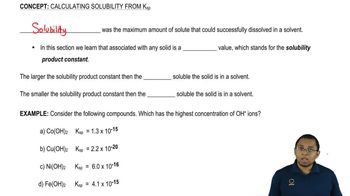01:30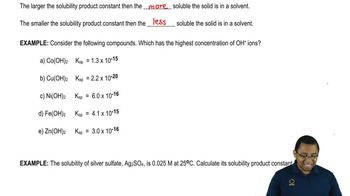01:5905:51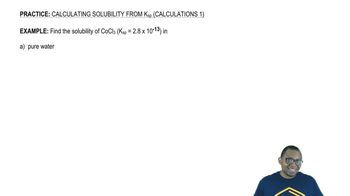05:21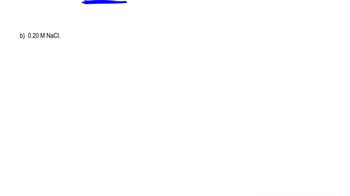04:11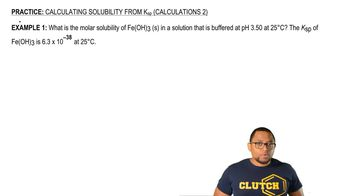04:5005:15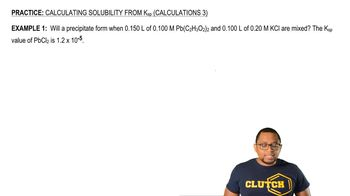07:1606:0503:47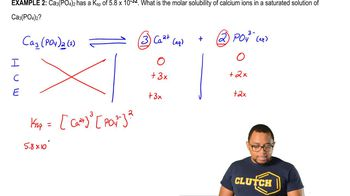04:1003:44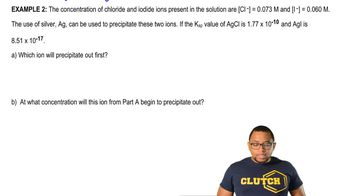03:36